Custom Search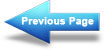Series-parallel DC circuits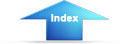Matter, Energy, and ElectricityRedrawing circuits for clarityPractice Circuit Problem
Figure 3-55 is a typical combination circuit. To make sure you understand the techniques of solving for the unknown quantities, solve for ER1.

Figure 3-55. - Combination practice circuit.It is not necessary to solve for all the values in the circuit to compute the voltage drop across resistor R1 (E R1). First look at the circuit and determine that the values given do not provide enough information to solve for ER1 directly.

If the current through R1 (IR1) is known, then ER1 can be computed by applying the formula: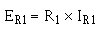The following steps will be used to solve the problem.

>The total resistance (RT) is calculated by the use of equivalent resistance.

Given: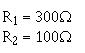Solution: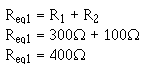Redraw the circuit as shown in figure 3-55(B).

Given:Solution:Solution: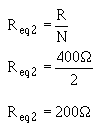Redraw the circuit as shown in figure 3-55(C).

Given:Solution:The total current (IT) is now computed.

Given:Solution:>Solve for the voltage dropped across Req2. This represents the voltage dropped across the network R1, R2, and R3 in the original circuit.

Given: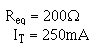Solution: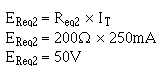>Solve for the current through Req1. (Req1 represents the network R1 and R2 in the original circuit.) Since the voltage across each branch of a parallel circuit is equal to the voltage across the equivalent resistor representing the circuit:

Given: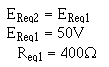Solution: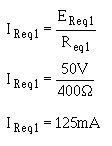Solve for the voltage dropped across R1 (the quantity you were asked to find). Since Req1 represents the series network of R1 and R2 and total current flows through each resistor in a series circuit, IR1 must equal IReq1.

Given: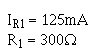Solution: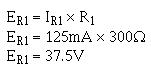Refer to figure 3-55(A). If the following resistors were replaced with the values indicated: R1 = 900Ω, R3 = lkΩ, what is the total power in the circuit? What is ER2?Integrated Publishing, Inc. - A (SDVOSB) Service Disabled Veteran Owned Small Business